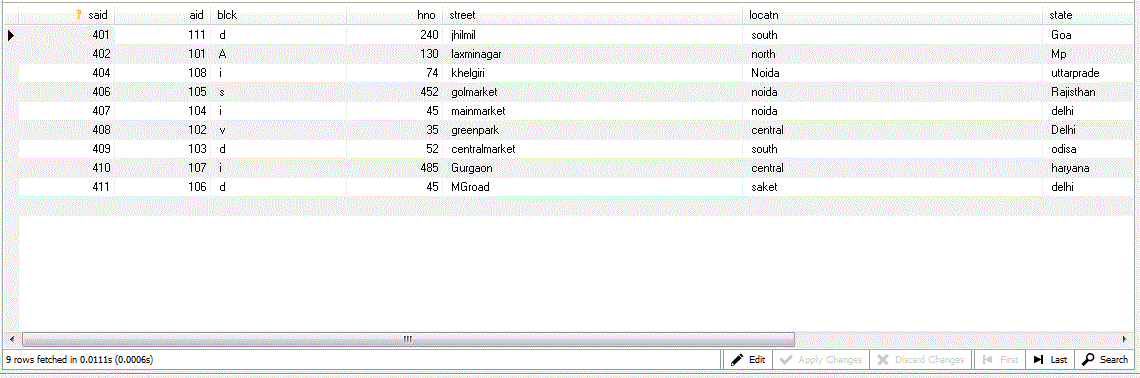# MySQL

Where in MySQL
 Previous Home Next

The Where command is used to filter the results according to the applied conditions. Here is the syntax of select statement.

```SELECT expressions
FROM tables
WHERE columnname operatorvalue;
```

There are some operators used in Where clause which is given below:

 Operator Description = Equal <> Not equal to(somtimes it may be written as !=) > Greater than < Less than <= Less than equal to >= Greater than equal to and Both conditions follow or Any one condition follow Between Within a Range

Here given some example which follow above conditions

In the following examples i have created a new table from the existing database of name school off name studadr as image given below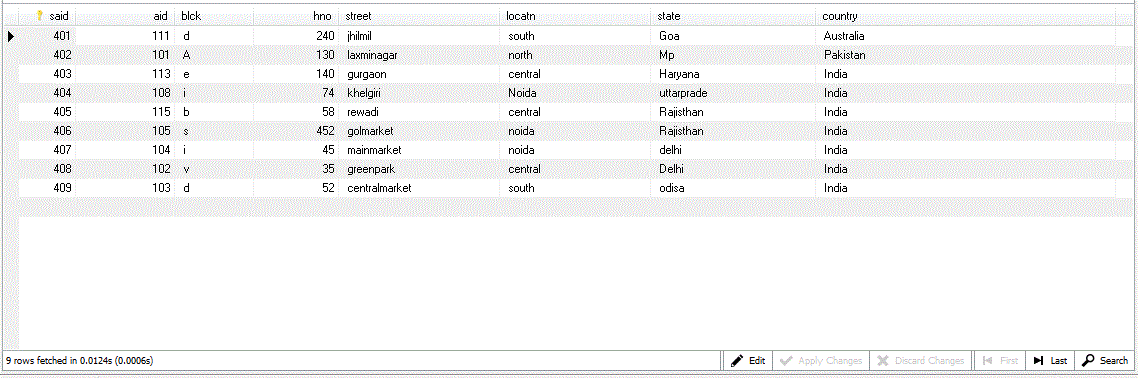### Example

```SELECT * 				//show all values
WHERE locatn = 'central' ;		//condition is given
```

### output become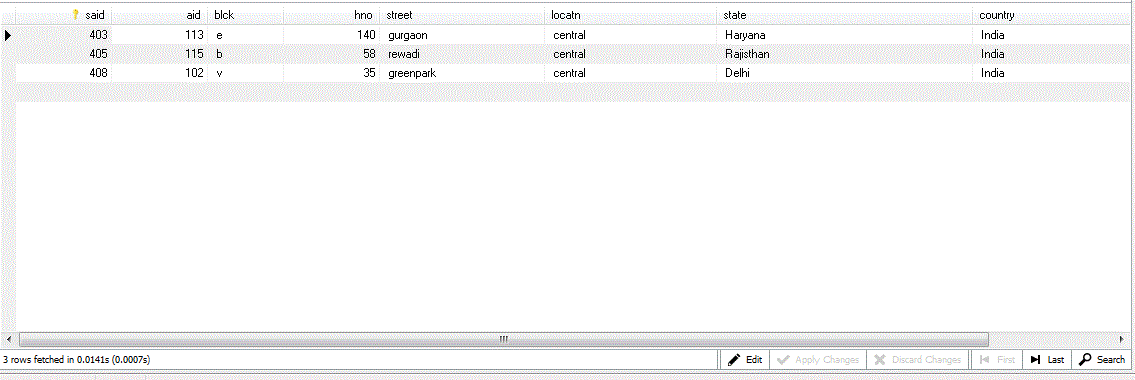### Example

```SELECT * 			//show all values
WHERE locatn != 'central' ;	//not equal to condition is given
```

### output become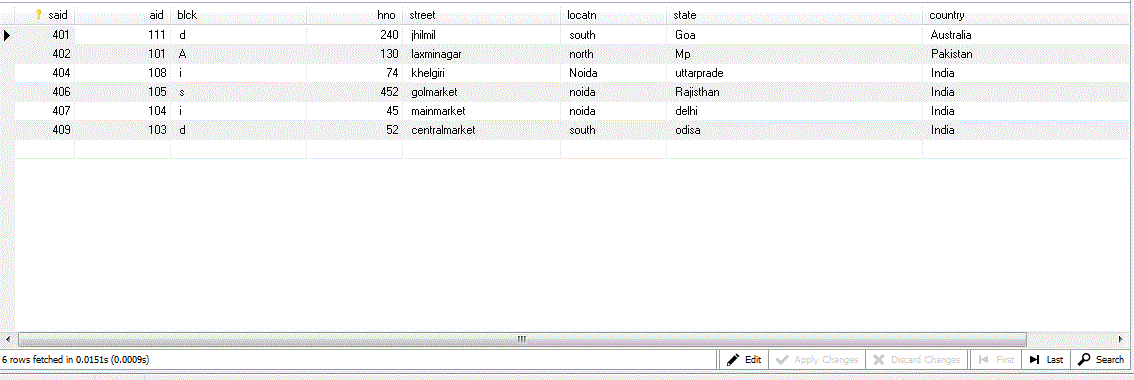### Example

```
SELECT * 			//show all values
WHERE aid  > = 108 ;		//greater than equal to condition is given
```

### output become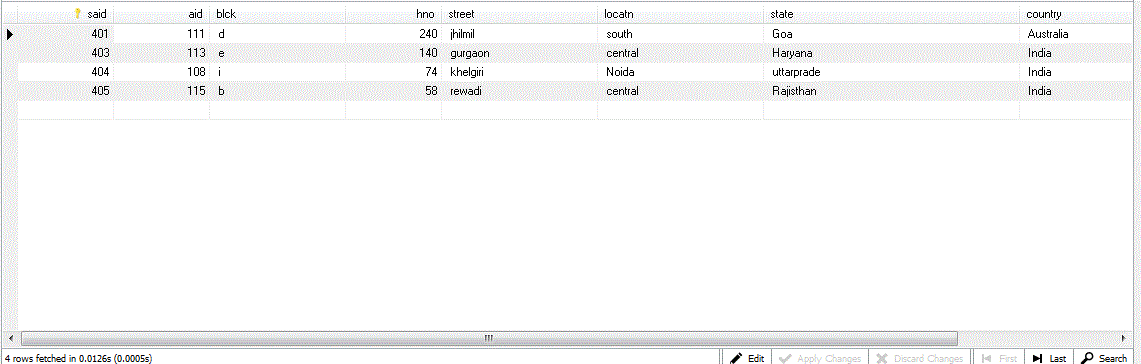### Example

```SELECT * 					//show all values
WHERE locatn ='noida' and state='delhi';	//AND conditions is given
```

### output become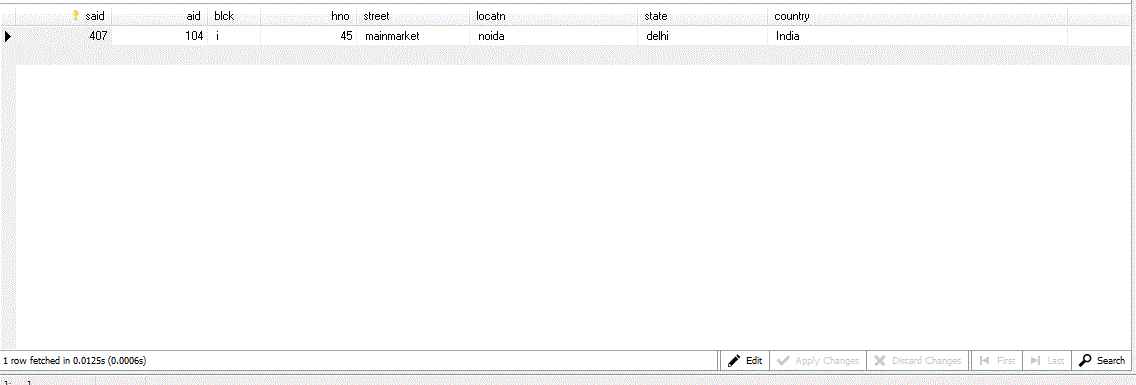### Note:

Both the conditions written on where clause should follow to get the output, if any one fails then no output will be shown.

### Example

```SELECT * 						//show all values
WHERE  locatn ='noida' OR state='delhi';;		//OR conditions is given
```

### output become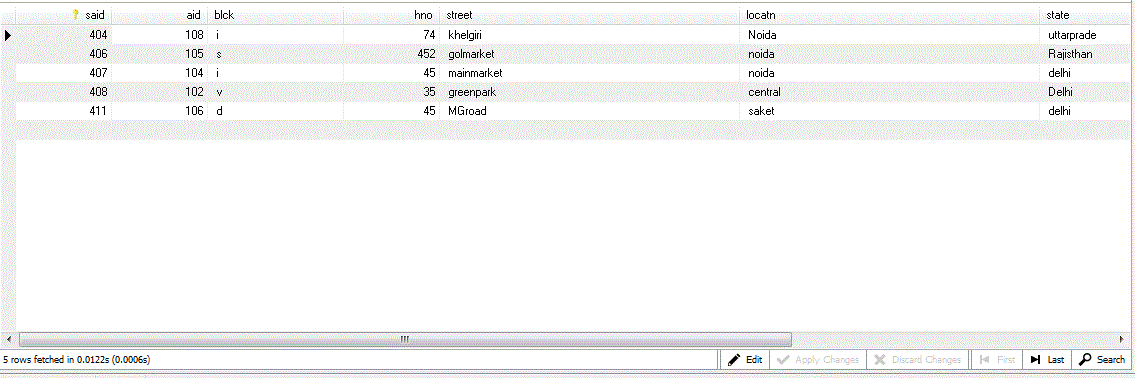### Note:

Any of the conditions written in where clause satisfy to get the output, if all the conditions fails then no output will be shown.

### Example

```SELECT *					//shoe all values